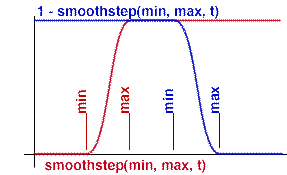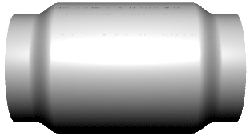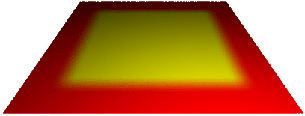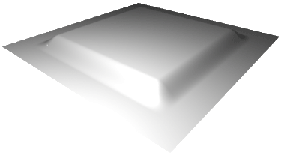### RSLUsing smoothstep

#### Introduction

The function `smoothstep()` is part of the maths library of shading language functions. Given three values, `min`, `max` and `input`, the function will return a number between 0 and 1 that represents the relationship of the `input` value to the `min` and `max` values.

If input is less than `min`, smoothstep() will return 0.
If input is equal to, or larger than `max`, smoothstep() will return 1.
If input is between `min` and `max`, smoothstep() will return a value (proportionately) between 0 and 1.0.

For example, suppose the min and max values are 0.3 and 0.6. Using the smoothstep function with input values of 0.4 and 0.8 we get output values of 0.35 and 1.0.

```
smoothstep(0.3, 0.6, 0.4);

smoothstep(0.3, 0.6, 0.8);
```#### Applying Smoothstep

As shown on the right the smoothstep function can be used to control the blending colors or a displacement. The code for the displacement shader is shown in listing 1.Figure 1 smoothcolor.slFigure 2 smoothbump.sl

Listing 1

 ```displacement smoothbump(float Km = 0.1, min = 0.3, max = 0.8) { float hump = smoothstep(min, max, t); normal n = normalize(N); P = P - n * hump * Km; N = calculatenormal(P); }```

#### Combining Smoothsteps - part 1

Often a shader must control a blending factor by smoothly increasing and decreasing an effect. For example, in the case of the displaced cylinder the min and max values might be used to define locations where a displacement is ramped-up then ramped-down.

The trick here is to notice that subtracting the values returned from the smoothstep() function from 1.0 has the effect of inverting its effect ie. the output values decrease from 1.0 to 0. A combined blending effect can be obtained by,

```blend = smoothstep(0.2, 0.3, t) * (1 - smoothstep(0.6, 0.7, t);
```Figure 3Figure 4 smoothcolor2.slFigure 5 smoothbump2.sl

#### Combining Smoothsteps - part 2

The double ramping seen in the previous section can also be achieved in two directions, say, in '`s`' and the '`t`'.

```blend = smoothstep(0.2, 0.3, s) * (1 - smoothstep(0.6, 0.7, s) *
smoothstep(0.2, 0.3, t) * (1 - smoothstep(0.6, 0.7, t);```Figure 6 smoothcolor3.slFigure 7 smoothbump3.sl